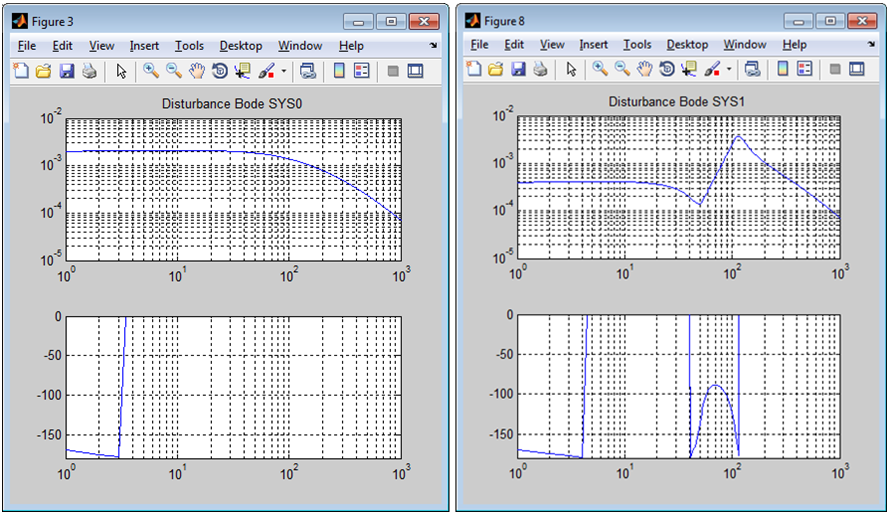Frequency Response Tuning Methods

﻿﻿﻿

The two most important criteria for servo systems are their ability to maintain the output at the setpoint and to reject external disturbances. Systems with a single degree of freedom can be optimized for either disturbance rejection or command following but not both. So a one degree of freedom controller with an excellent settling time will likely be susceptible to an external disturbance. A two degree of freedom controller, on the other hand, can be designed for both command following and disturbance rejection.

Frequency response analysis provides valuable information that can be used to understand and optimize the system performance. This article will explain four frequency response measurements to that can provide the information needed to analyze the command following and disturbance rejection performance of a control system.

Open and closed loop measurements are the most commonly performed frequency response measurements today.  These measurements alone do not provide the full picture especially when it comes to disturbance rejection.  In systems where pole zero cancelation occurs the open loop response can produce misleading results.  To fully understand disturbance rejection the disturbance measurement is needed.

The Measurements

There are several advantages to analyzing a control system in the frequency domain. Variable frequency sinusoidal signal generators and precision instruments for measuring magnitude and phase are readily available while it is considerably more difficult to measure the time response of a system. It is usually much easier to determine the stability of feedback control systems in the frequency domain. It’s also worth noting that the time response and frequency response of the system are related through Laplace transformation so time domain performance measures can be converted to frequency measures and vice versa.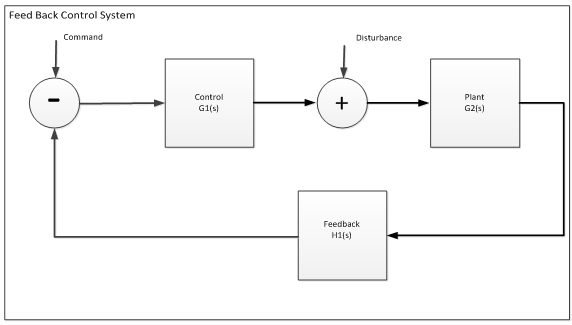Figure 1: Feedback control system

Figure 1 shows a basic feedback control system. A transfer function for each of the blocks in the feedback control system is defined as a function of s in the frequency domain. There are 4 basic measurements that should be performed on a system.  They are Open Loop, Closed Loop, Disturbance Rejection and Q parameter (control output ). The transfer function for each basic frequency response tuning measurement is provided in the table below.

Open Loop G(s)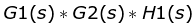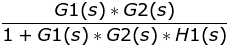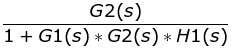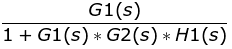Table 1: Transfer function description of frequency response tuning measurements

• The open loop transfer function determines the stability of the system. Open loop response is measured by disconnecting the feedback loop and measuring from the command to the output of the feedback device.
• The closed loop transfer function determines its command following capability. Closed loop response is the relationship of input to output of the control variable. For example for a closed loop position control system closed loop response defines how well the motor position follows the desired position command.
• The disturbance transfer function evaluates the ability of the system to reject disturbances.   Disturbance is measured by injecting random noise into the disturbance node and measuring the output.
• The Q parameter is an indication of sensitivity to noise.  The Q parameter is measured by injecting noise into the command node and measuring the control block output.

Measurement Methods and Bode Plots

Frequency response measurements are performed by exciting the input at different frequencies. An excitation signal for a given range of frequencies is applied to the input while measuring the amplitude and phase angles of the outputs. One common mode of excitation is random, where one signal is applied that contains the all frequencies of interest. The other common excitation mode is swept sine excitation where the system is excited at each specific frequency of interest. The result of swept sign excitation is sinusoidal output with the same frequency as the input but usually with a different magnitude and phase. The frequency response of the system determines the magnitude and phase of the sinusoidal output.

While there are several different ways to describe the system frequency response this article will focus on the Bode plot. The there are two types of Bode plots: amplitude vs. frequency and phase vs. frequency. The frequency response of all the measurements defined in this article can be expressed in Bode plots.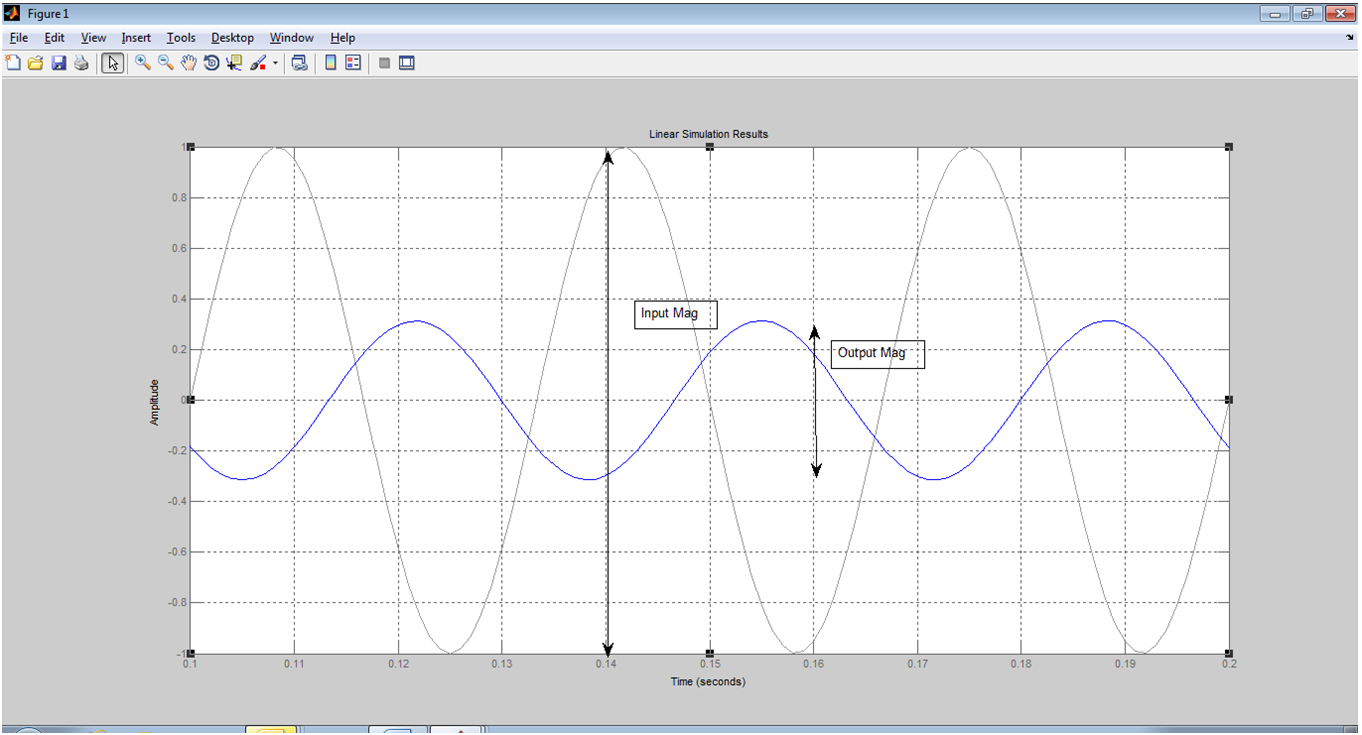Figure 2: Bode amplitude plot

The Bode amplitude plot shows the amplitude ratio, the amplitude of the output sinusoidal curve divided by the amplitude of the input sinusoidal curve, as a function of frequency.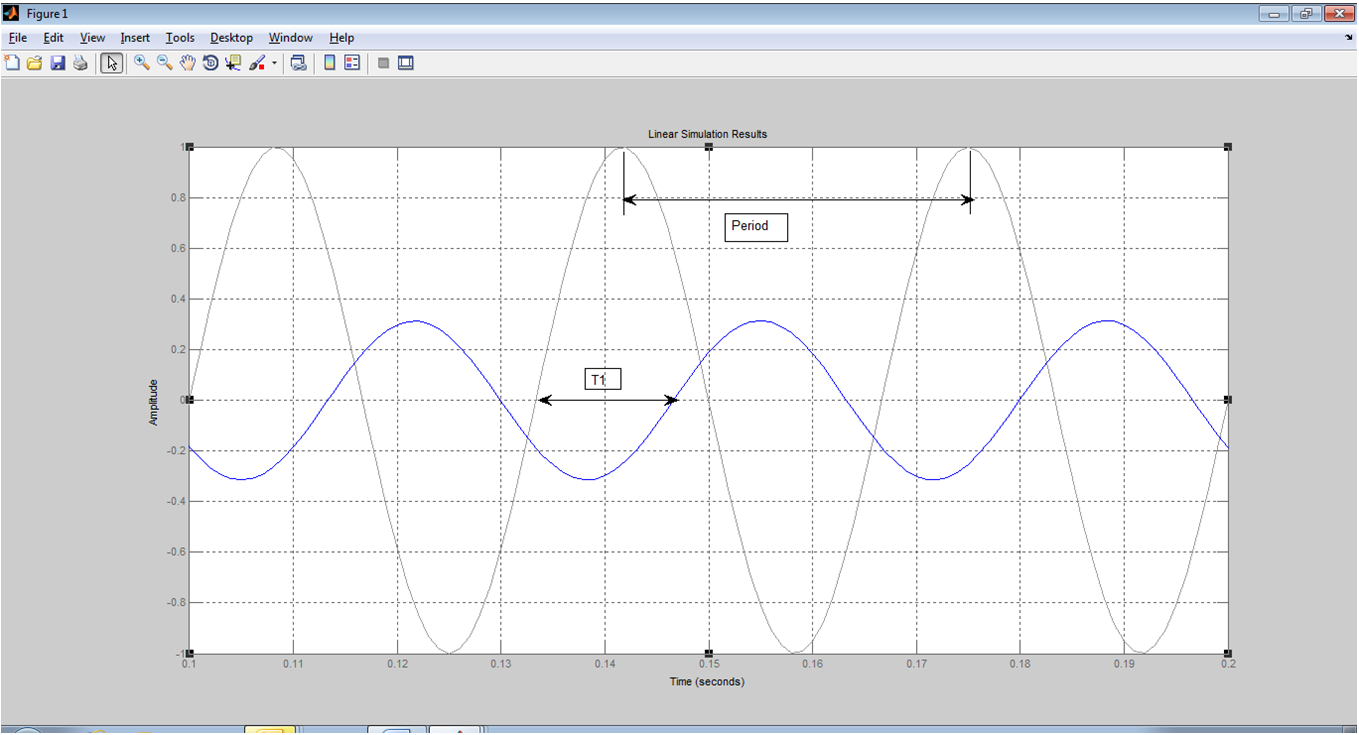Figure 3: Bode phase plot

The Bode phase plot shows the ratio between the output and input phase shift as a function of frequency. The phase shift is measured across a range of frequencies and each value is plotted as a function of frequency.

Open loop measurement measure of stability

The gain crossover frequency is the point on the Bode amplitude plot where the gain is equal to 1 or 0 dB. A good system has a clear crossing at the gain crossover frequency. For an open loop system, the gain increases at frequencies below the gain crossover frequency and the gain drops at frequencies above the gain crossover frequency. For a closed loop system, the gain is near 1 for frequencies below the gain crossover frequency and drops for frequencies above the gain crossover frequency. A high gain in particular is needed at frequencies lower than the gain crossover frequency.

The gain margin is the difference between the amplitude curve and 0 dB at the point corresponding to a frequency that generates a phase of -180 o, the phase crossover frequency.  Gain margin defines the amount of gain change that can occur before instability occurs. The phase margin is the difference in phase between the phase curve and -180o at the point corresponding to a frequency that generates a gain of 0 dB, the gain crossover frequency. For example, if the phase at the crossover frequency is -135 then phase margin is 180o – 135 o = 45 o. At least 45 o of phase margin is recommended. Phase margin determines the amount of phase lag that can occur before instability occurs.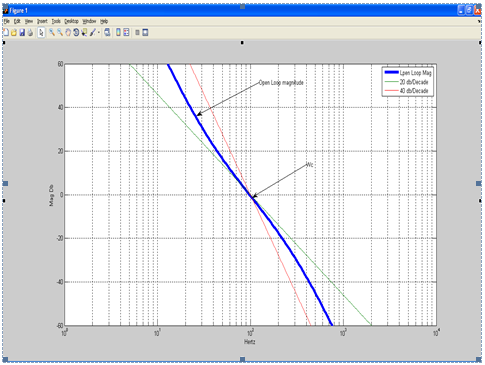Figure 4: Gain crossover frequency shown on Bode amplitude plot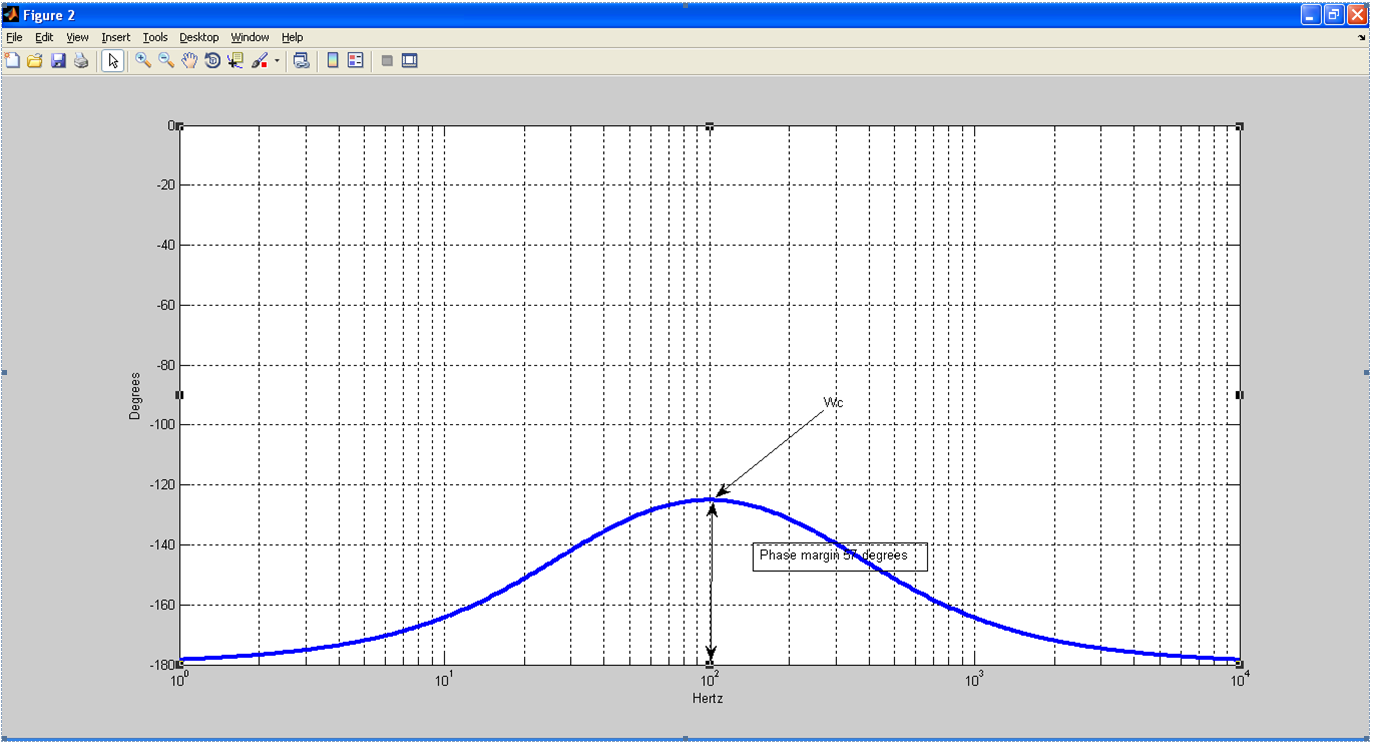Figure 5: Bode phase plot showing phase margin

Figure 5 shows an open loop Bode plot with frequency on the x axis and amplitude on the y axis. The gain crossover frequency is 100 Hz in this system. The crossover frequency provides an indication of the ability of the system to respond to follow a changing input. A system that controls a box moving on a belt conveyor probably does not need a lot of bandwidth, however, a wire bonder would require much higher bandwidth. For example, if you have a system that needs to be able to move to a new setpoint in 100 ms, then you need a crossover frequency in the range of 20 Hz or higher.

The relative stability of the system, in other words the amount of damping, is a function of open loop phase margin at crossover. The difference between the phase and -180 o at 100 Hz, which has already been determined to be the crossover frequency, is 55o so this is the phase margin.

A control system is unstable if the open-loop frequency response exhibits an amplitude ratio of 1 and a phase shift of -180 degrees at the crossover frequency.

A feedback control system always operates in closed loop. The open loop gain and phase margins are measures of the robustness and stability of the system when operating in closed loop. The larger the phase and gain margins, the more tolerant the system will be to component variations.

As a general rule, increasing the gain increases the amplitude ratio and reducing the gain reduces the amplitude ratio but does not affect the phase angle. A change in the time delay of the system has an effect on the phase angle but does not affect the amplitude ratio.

Systems with nonlinearities and disturbances of relatively large magnitudes require larger phase margin and gain margin values. Phase margin values typically range from 300 to 55o. Industrial control designers typically choose gain margin values of 2 or higher to ensure robustness.

Controllers can perform both command following and disturbance rejection tasks, however, the controller generally needs to be tuned for optimal performance in one role or the other. A setpoint tracking controller will tend to overreact in response to a sudden change and cause the process variable to oscillate. On the other hand, a controller tuned primarily for disturbance rejection will take a relatively longer period of time to implement a setpoint change.

The magnitude of the crossover frequency determines the rise time. The higher the crossover frequency, the faster the rise time. The phase margin determines amount of damping in the system which in turn determines the overshoot. If a step response is applied to the input of an under-damped closed loop system, the system will overshoot and eventually settle to the command value. If the system is over-damped, it will gradually creep to the setpoint value. If it is critically damped, it will reach the set point with minimum overshoot and settling time.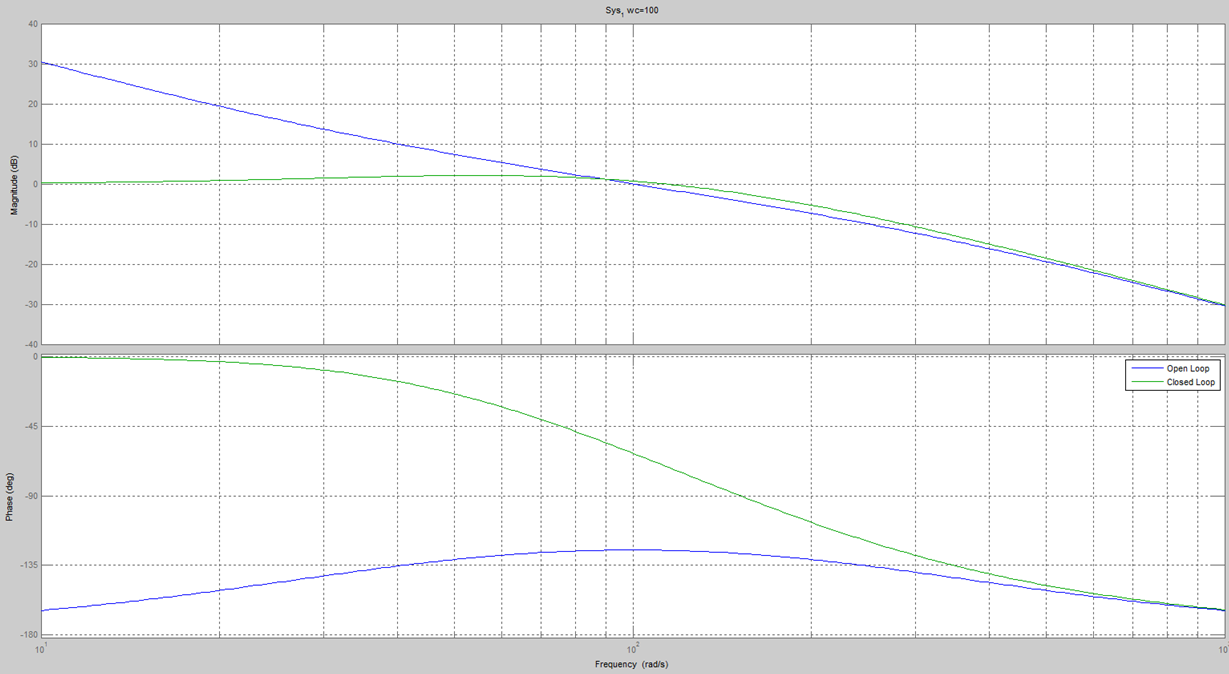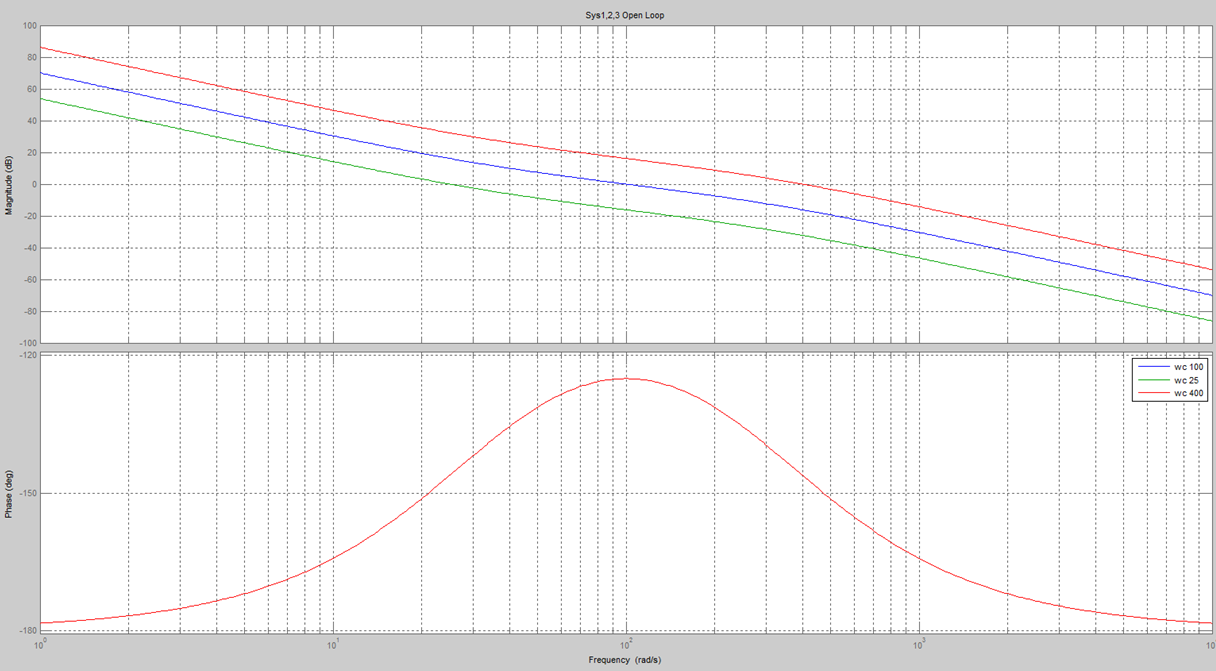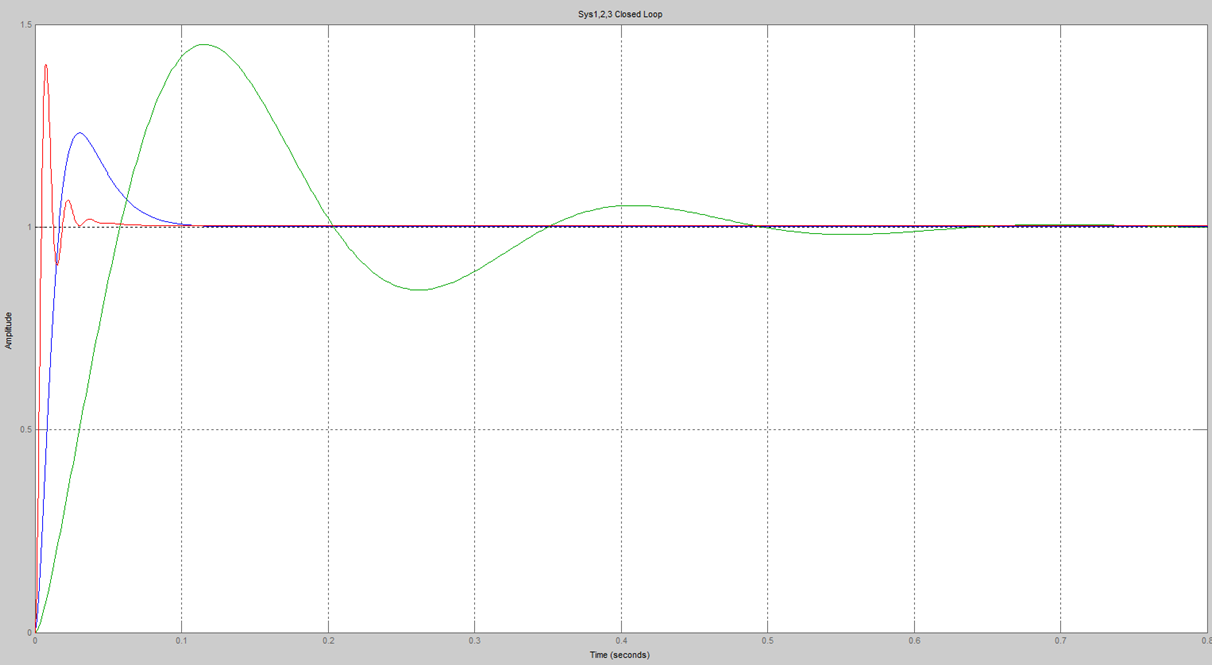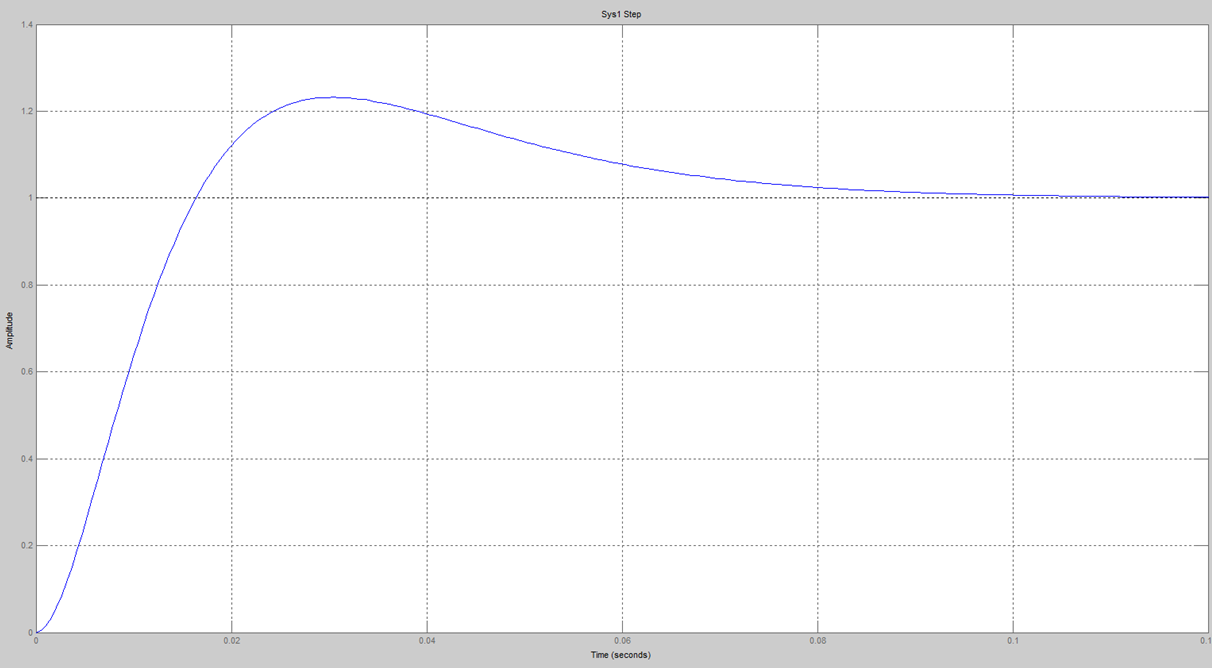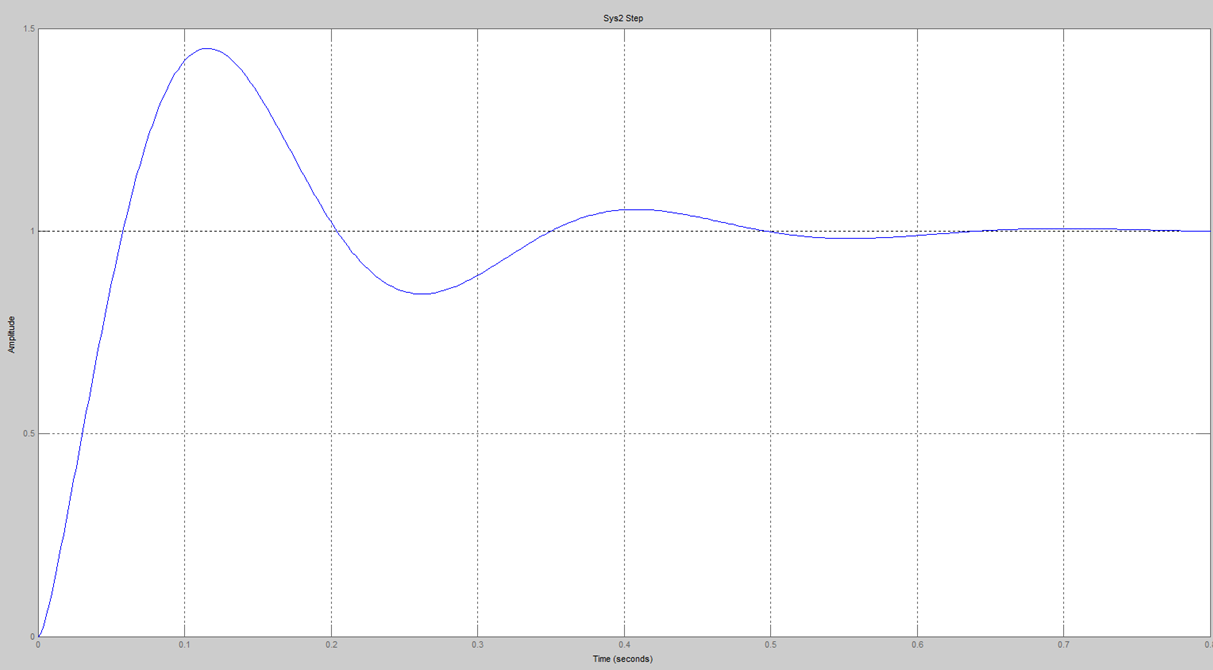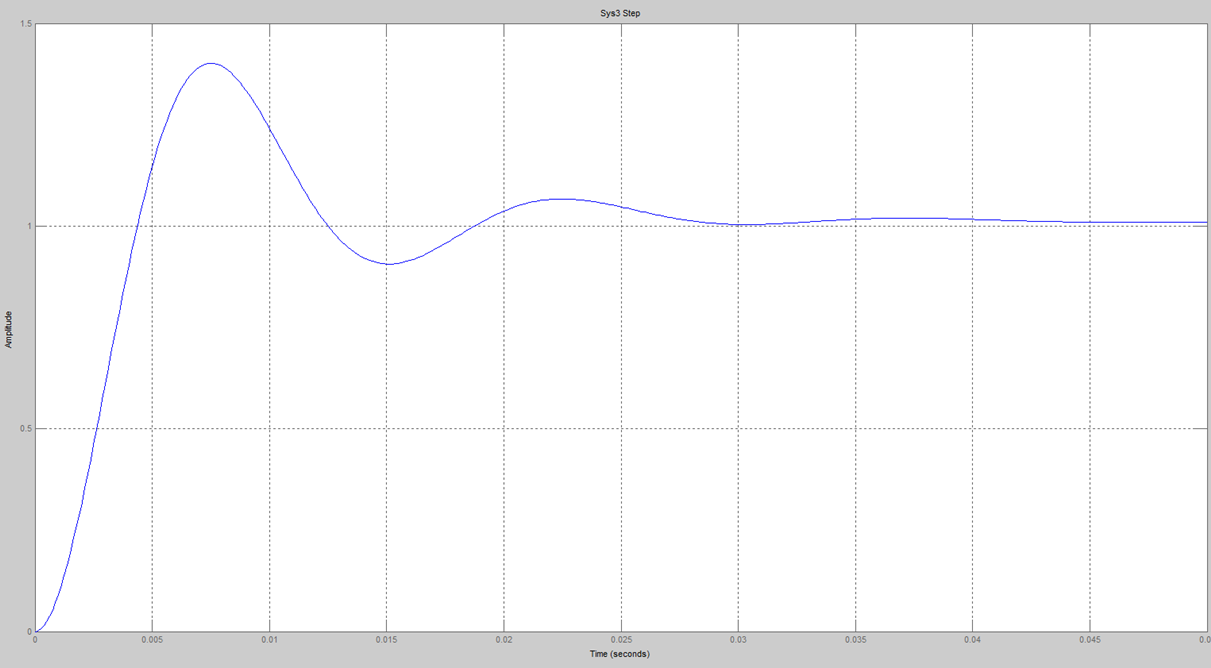Disturbance Measurement

One of the key issues in any industrial control system is its robustness with respect to perturbations. Disturbances can range from torque disturbances to mechanical shocks. Open loop analysis by itself does not provide an accurate picture of the disturbance rejection capabilities of a control system. For example, you might have two linear motors providing stable operation on the same track. When one motor moves its torque ripple generates a disturbance on the motor standing still. The stationary motor will move due to the disturbance in the worse case. If the frequency of this disturbance matches a mechanical resonance on the motor then it will shake. The disturbance measurement will determine the system stiffness and how well it can reject external disturbance

A control system should be designed to account for the possibility that change will occur. For example, the bearings in the motor might wear and cause an increase in friction and the load driven by the motor might also change over time. One approach to address this question is to estimate the effect of a change in the open loop gain on the closed loop gain. A system where the closed loop gain is not very sensitive to changes in the open loop gain will be more stable over time.

How Disturbance Measurements Detects Pole Zero Cancelations

Open loop response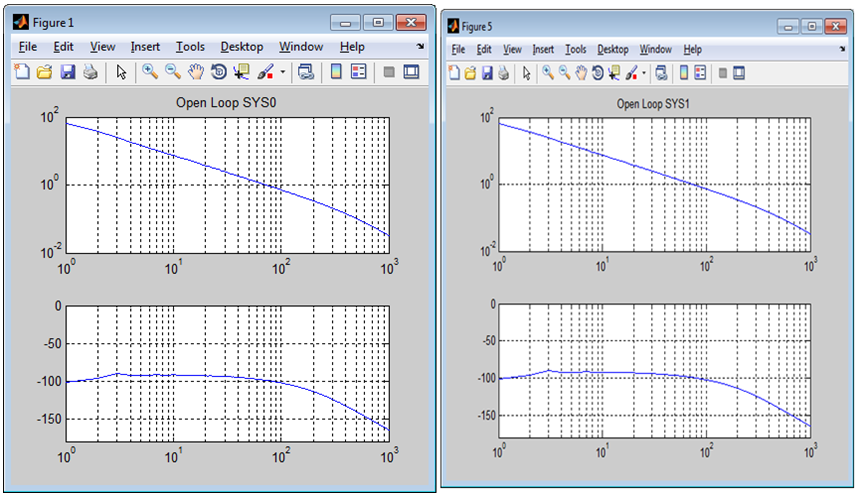Closed loop response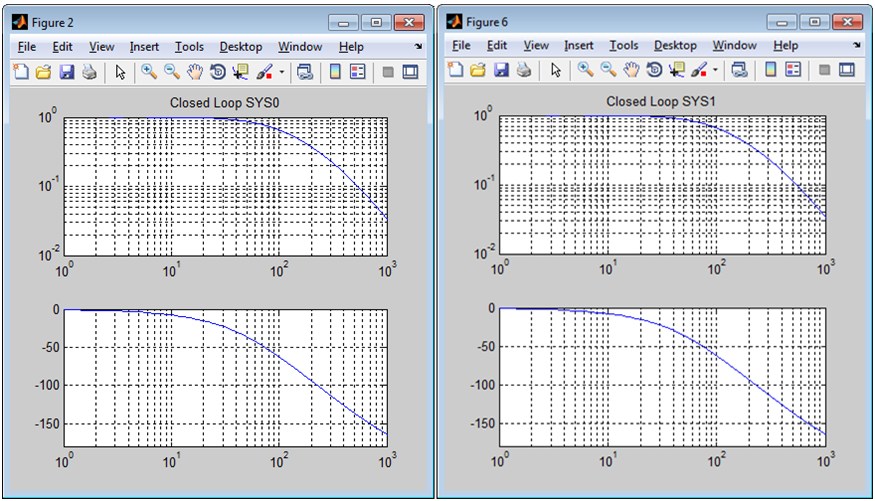Command step response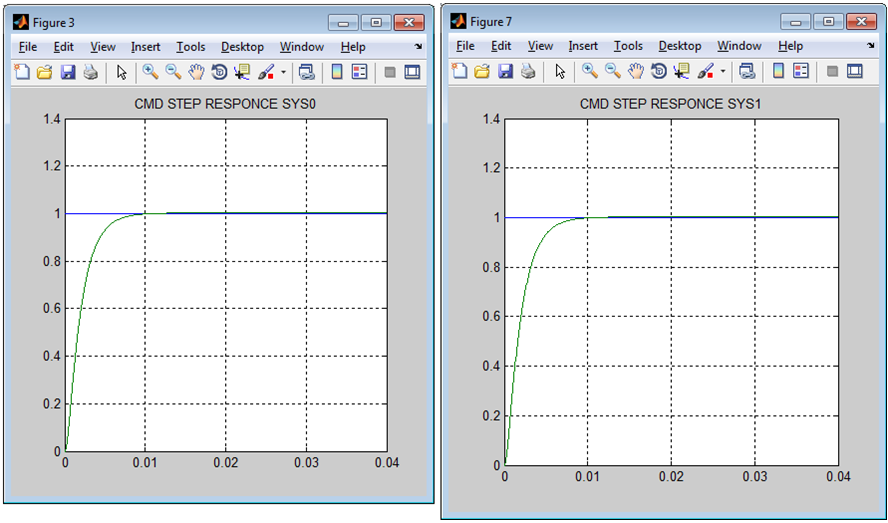Disturbance input step response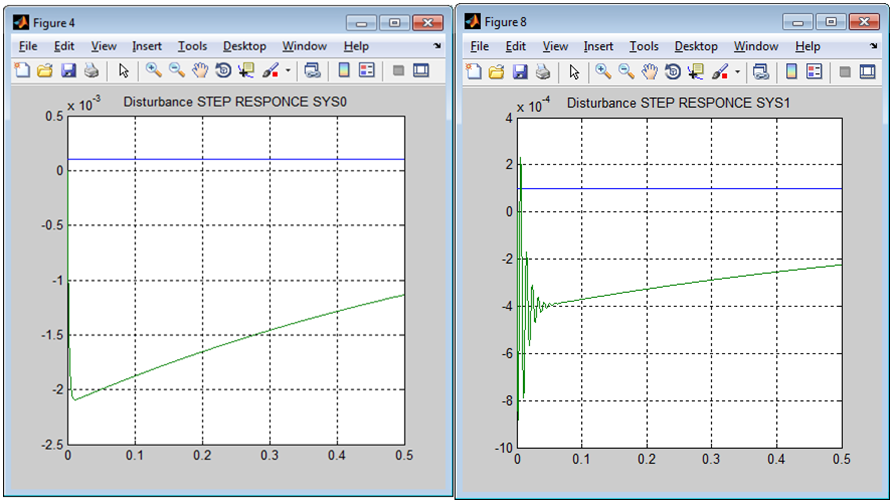Disturbance Bode plots